×#### Thank you for registering.

One of our academic counsellors will contact you within 1 working day.

Click to Chat

1800-1023-196

+91-120-4616500

CART 0

• 0

MY CART (5)

Use Coupon: CART20 and get 20% off on all online Study Material

ITEM
DETAILS
MRP
DISCOUNT
FINAL PRICE
Total Price: Rs.

There are no items in this cart.
Continue Shopping• Complete JEE Main/Advanced Course and Test Series
• OFFERED PRICE: Rs. 15,900
• View Details

```Chapter 18: Binomial Theorem – Exercise 18.2

Binomial Theorem – Exercise – 18.2 – Q.1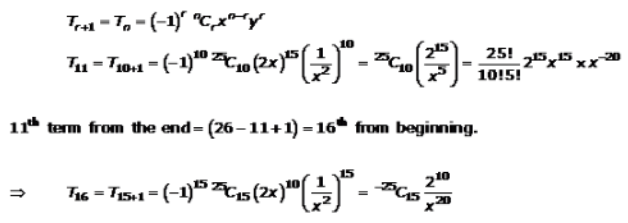Binomial Theorem – Exercise – 18.2 – Q.2Binomial Theorem – Exercise – 18.2 – Q.3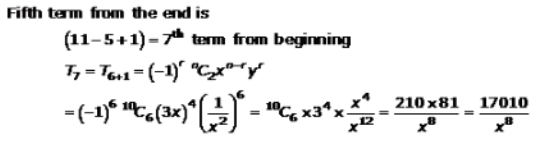Binomial Theorem – Exercise – 18.2 – Q.4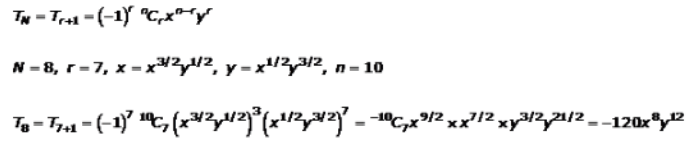Binomial Theorem – Exercise – 18.2 – Q.5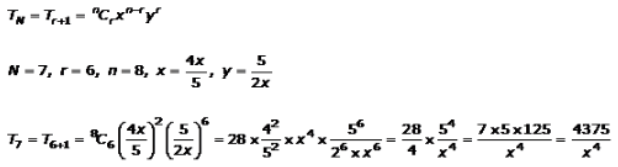Binomial Theorem – Exercise – 18.2 – Q.6Binomial Theorem – Exercise – 18.2 – Q.7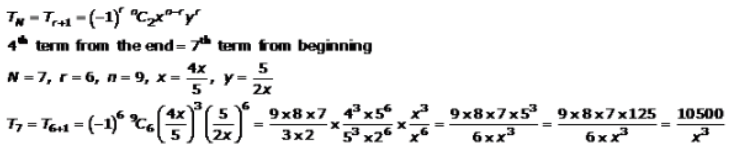Binomial Theorem – Exercise – 18.2 – Q.8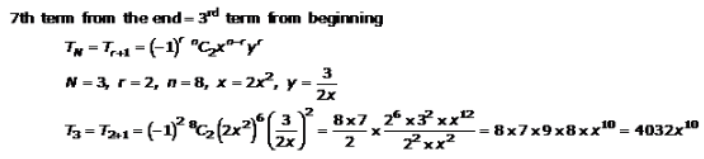Binomial Theorem – Exercise – 18.2 – Q.9(i)Binomial Theorem – Exercise – 18.2 – Q.9(ii)Binomial Theorem – Exercise – 18.2 – Q.9(iii)Binomial Theorem – Exercise – 18.2 – Q.9(iv)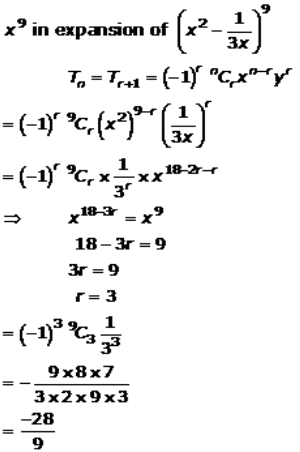Binomial Theorem – Exercise – 18.2 – Q.9(v)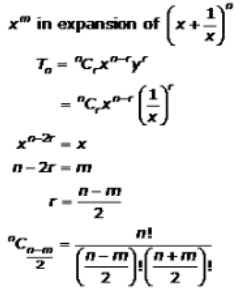Binomial Theorem – Exercise – 18.2 – Q.9(vi)Binomial Theorem – Exercise – 18.2 – Q.9(vii)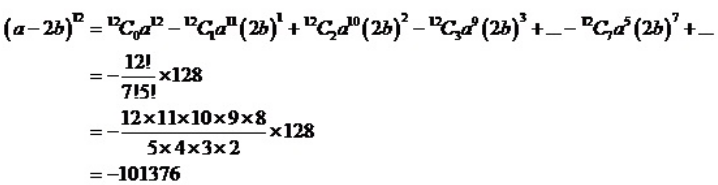Binomial Theorem – Exercise – 18.2 – Q.9(viii)Binomial Theorem – Exercise – 18.2 – Q.10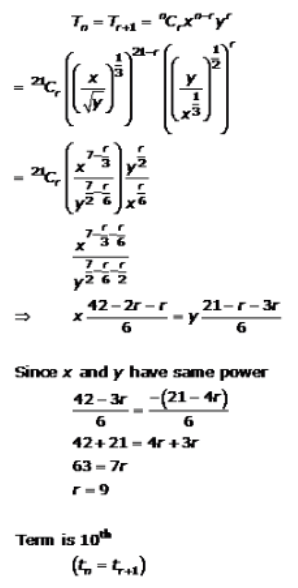Binomial Theorem – Exercise – 18.2 – Q.11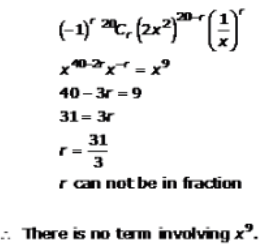```### Course Features

• 728 Video Lectures
• Revision Notes
• Previous Year Papers
• Mind Map
• Study Planner
• NCERT Solutions
• Discussion Forum
• Test paper with Video Solution